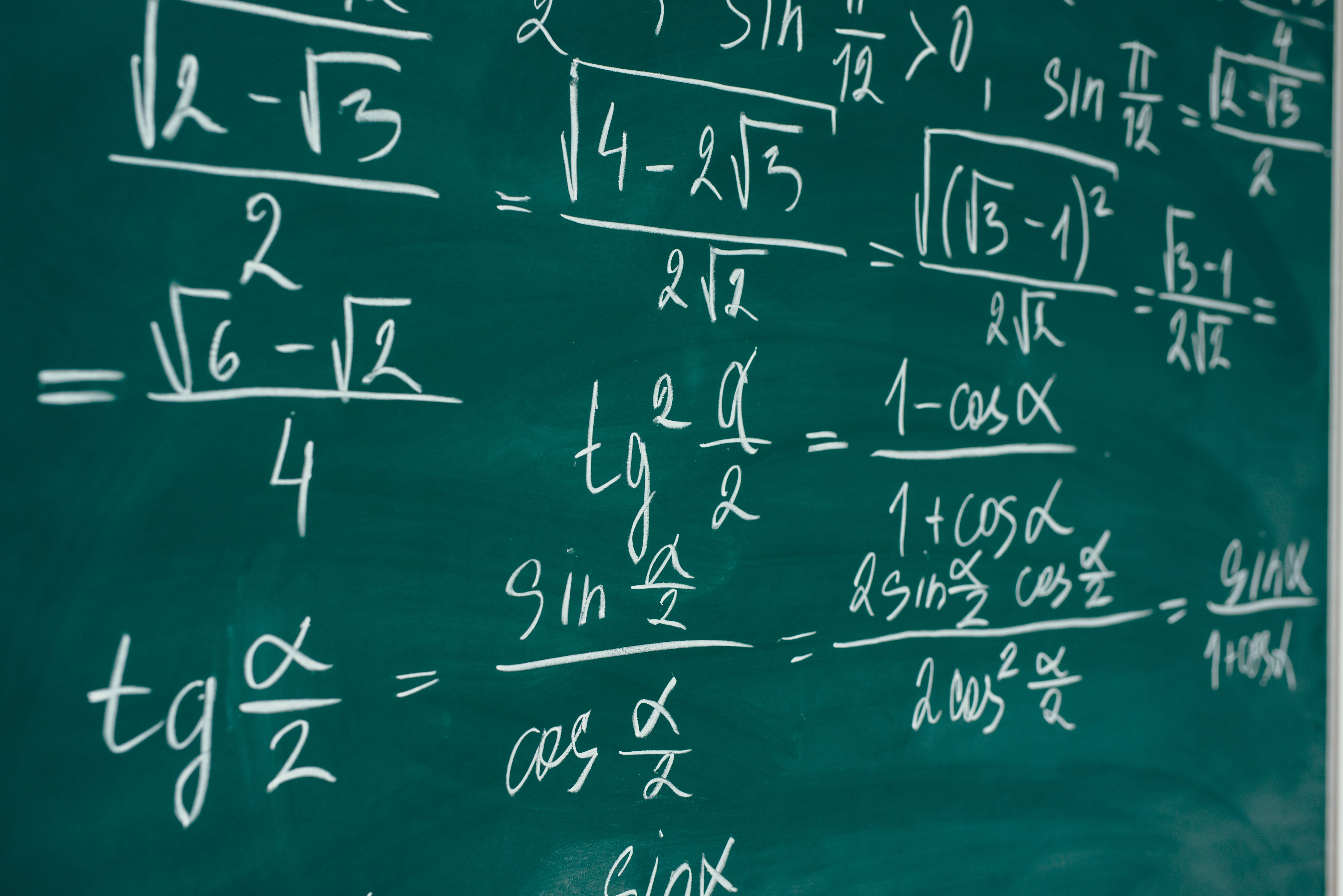# Greatest Common Factors In Algebra

Teachers have finished reviewing pre-algebra topics, making sure everyone remembers what they learned last year.## By now, algebra students in Newport Beach have watched summer vacation fall behind them.

Teachers have finished reviewing pre-algebra topics, making sure everyone remembers what they learned last year.  At this point, your student may have been doing fine in their math class.  But with the introduction of new topics, some algebra students start to fall behind and need the assistance of a tutor.

One Algebra subject that Newport Beach students will begin covering in-depth is factoring.  You probably remember various methods of factoring from your own math class, and every algebra teacher has a preferred method.  Your student may come home talking about the “x” method, drawing a box, a bug, a caterpillar, two parentheses…each of these methods will work, and just take time to practice and perfect.  But before these more complicated factoring problems, algebra students in Newport Beach will be working on GCF, or Greatest Common Factor.

A Greatest Common Factor is a number or variable that can be divided out of every piece of an algebraic expression.  An algebra tutor might start with a simple example, such as:

Find the GCF of 2x + 4

In this case, both 2x and 4 can be divided by two.  The GCF is two.  The factored expression is:

2(x + 2)

A slightly more complicated example is the following:

Find the GCF and factor 15xy – 40x + 35xz

There are three terms to look at.  A student may be able to see the common GCF right away.  If not, an algebra tutor will ask a student to find the factors of each piece, then see what’s in common.  In this example:

Factors of 15xy = 3, 5, 15, x, and y

Factors of 40x = 2, 4, 5, 8, 10, 20, 40, and x

Factors of 35xz = 5, 7, 35, x, and z

Then, a tutor will ask a student to circle the factors that all three pieces have in common.  Check and see if you can find the factors above!  Did you find 5 and x?

The GCF is 5x.  The factored expression is:

5x(3y – 8 + 7z)

With practice, finding the GCF will become easier, and an algebra student in Newport Beach will be able to move on to harder factoring problems.

Getting Help in Algebra

If your student is having difficulty understanding algebra, Firefly Tutors offers excellent algebra tutoring to increase understanding and improve grades.

We have outstanding algebra tutors that will come to your home in Newport Beach,

Huntington Beach, Irvine, and Costa Mesa as well as tutors for other subjects throughout Orange County.